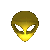## Converting between Unreal Units and other units-- Rotations and Rotationrates --

Following is a program/script with which you can convert values between and to Rotational Unreal Units (RUU). So for example x degrees is n degrees when converted to RUUs.
I've written another page which contains a script with which you can convert between Unreal Unit lengths and other units.

How to use this document

To convert between units, first select which unit you want to convert.
Next, select the unit you want to convert the value to and enter the value in the blue text box.
Hit the Convert button to convert the value and the answer will appear in the other text box.
Thanks to a script (which I didn't write) the answer will be automatically copied to the clipboard. =)
If you want to make an object rotate at a certain rate, you use the standard rotation and divide this by 1 second. For example:

 1 rotation per second (1 Hz) = 360°/sec = 2 Pi/sec = 65536 RUU 2 rotations/sec (2 Hz) = 720°/sec = 4 Pi/sec = 131072 RUU 0.5 Hz = 180°/sec = 1 Pi/sec = 32768 RUU

And you enter this value (RUU) into the RotationRate in an object's properties under movement. Easy, huh? :)